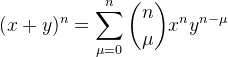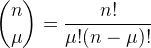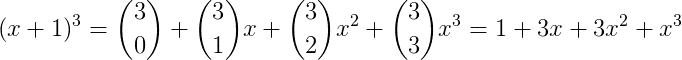# What is the simplest way of selecting the last N terms of a polynomial?

If you have a polynomial like (1+x)^6 = x^6+6 x^5+15 x^4+20 x^3+15 x^2+6 x+1, What function would you use to pick only the last N terms? For instance, for N=3 pick x^6+6 x^5+15 x^4

I've being using sum of a binomial times something, but was wondering if there is anything more simple.

Thanks!

Stephen Tashi
What function would you use to pick only the last N terms?
What do you mean by "to pick"? Can you state your question mathematically?

Here:wherefor example:^ I think he might just mean an (ordered) set of terms from the polynomial. The order is established by listing the coefficients in descending order of their degree.

I think what you want isn't so complicated. It's as follows: if $p \in \mathbb{R}\left[x\right]$ is a polynomial with degree n, i.e. $p(x) = {p_n}{x^n} + {p_{n - 1}}{x^{n - 1}} + ... + {p_1}x + p_0$, define the function $F_k: \mathbb{R}\left[x\right] \rightarrow \mathbb{N}^k$ by ${F_k}(p) = (p_n, p_{n - 1}, ..., p_{n - k})$. This "gets" the first (or if you want, last) k coefficients, which is really all you need to define a polynomial.

Last edited:
Here:wherefor example:Exactly! This is the function I've being using. What I wonder is if there is a more simple version of it.

Thank you very much anyways!

I think it's the simplest way. May be, if you don't want to deal with combinatorics, use "[URL [Broken] triangle[/URL]

Last edited by a moderator:
That only works for polynomials which can be expressed in the form (1 + x)n, though ...